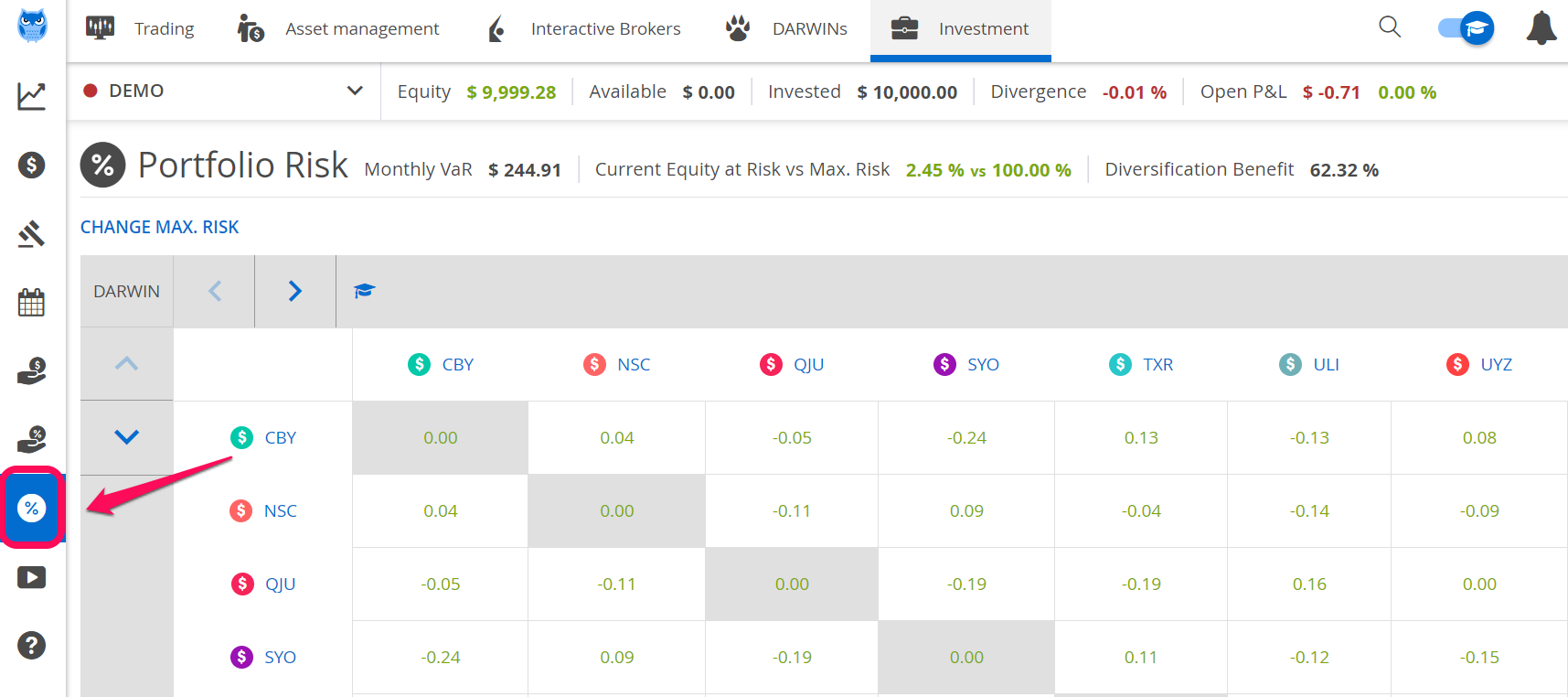1. Darwinex Docs
2. World of Darwinex
3. Darwinex Metrics and Graphs

# What is Value at Risk (VaR)?

## The VaR or Value at Risk is a way of measuring the risk of an investment which answers the questions how much you might lose, how likely it is, and over what timespan.

An investment having a maximum monthly Value at Risk of 6.5% with a 95%  confidence level might expect to lose 6.5% or more one month out of 20 months, or 5% of the time.

The flip side of this is that, 95% of the time or 19 months out of 20, this same investment will make more than -6.5%.

### Risk describes what could happen in the future

Risk is a forward-looking measure, unlike drawdown which describes what did happen in the past.

Since risk describes what could happen to your money in the future, it's related to a target horizon.

At Darwinex this horizon is 1 month.

### What does VaR mean?

Value At Risk (VaR) determines the potential for loss in a financial asset, the probability of occurrence for the defined loss, and the timeframe.

In Darwinex we use a monthly VaR with a 95% confidence level, therefore it estimates, given normal market conditions, how much an investment might lose in a month with 95% probability.

In this chart, you can see how return on an investment evolves over time.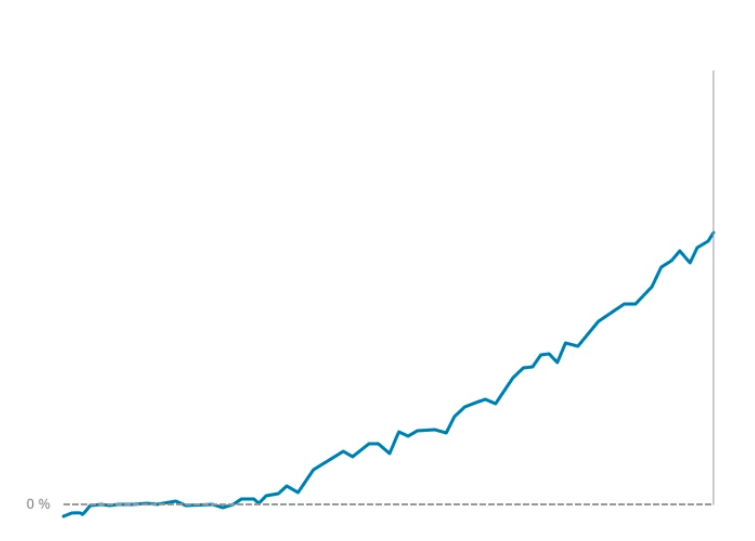What could happen to the return of this investment in the next month?

In order to respond to this question, different scenarios get projected taking into account both historical data and thousands of Monte Carlo simulations matching both the risk and investment style.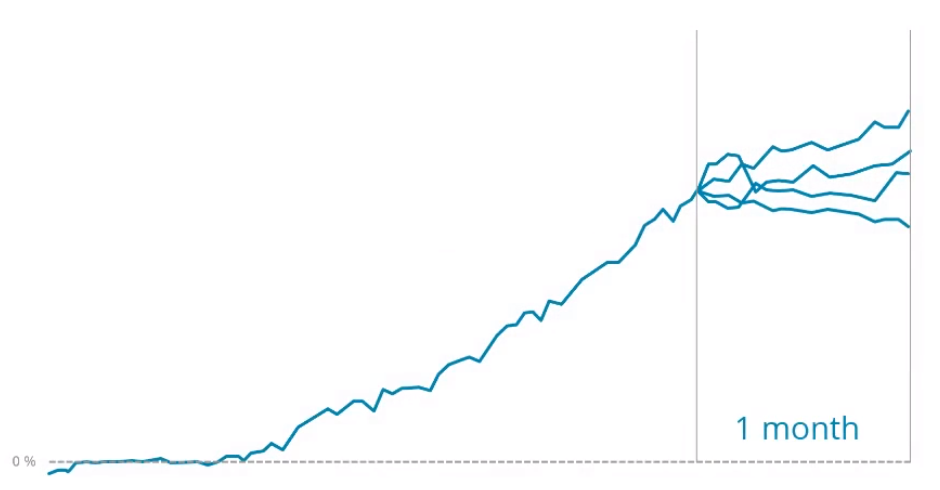Projections for investments with lower volatility will present lower volatility than projections for investments with higher volatility which will result in more dispersed scenarios.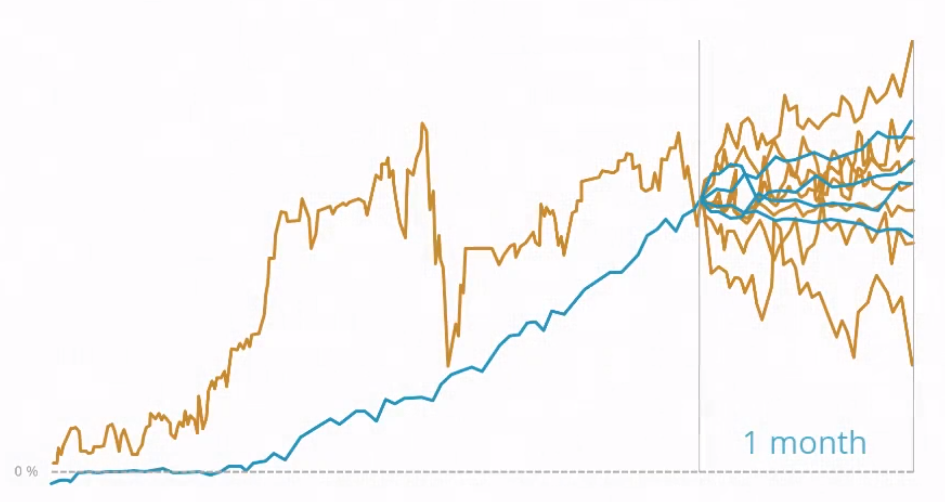These return projections are plotted on a distribution graph.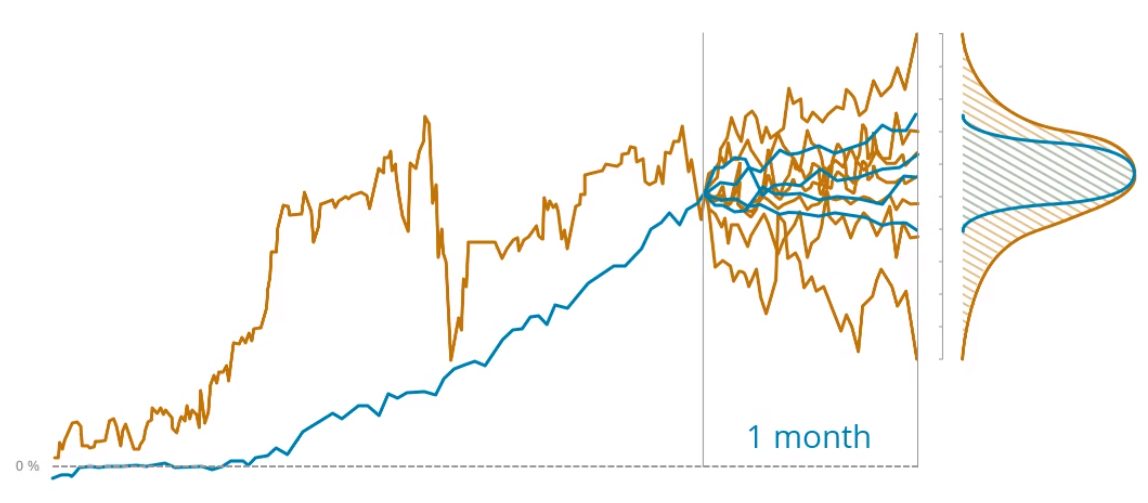95% VaR will be situated at the 95% percentile of the distribution graph.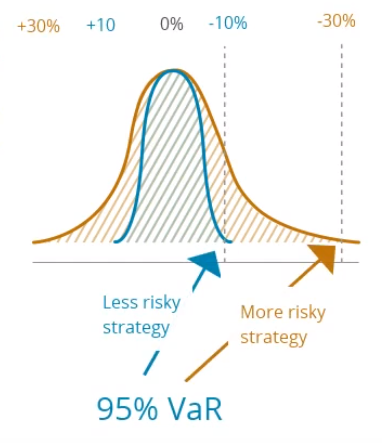As an example, an investment having a monthly Value at Risk of 10% might expect to lose 10% or more 1 month out of 20 or 5% of the time.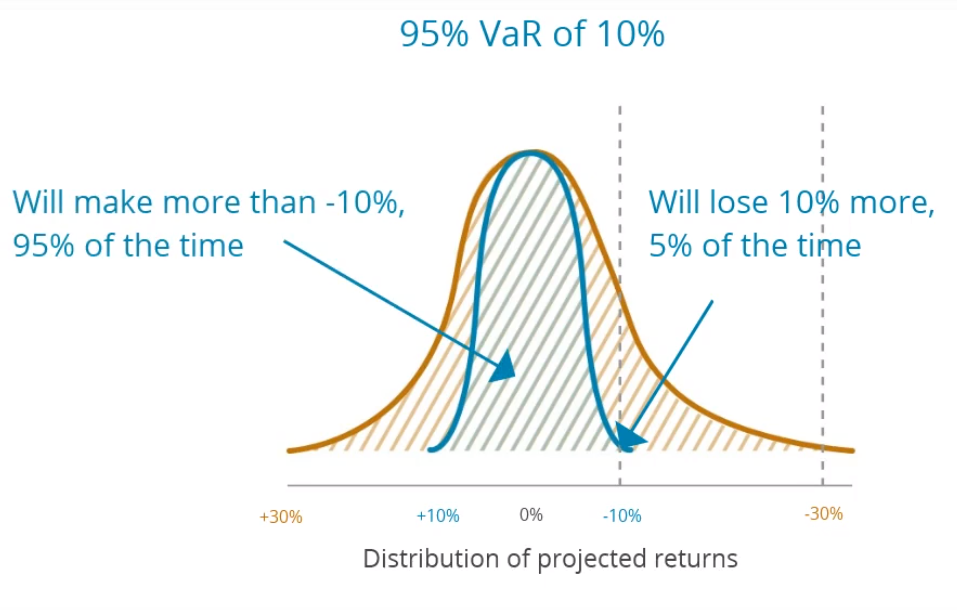### Probability

It is impossible to have 100% accuracy when it comes to making predictions about the future. Therefore, it's common to work with confidence level of 90%, 95%, or even 99%.

The higher the confidence interval is, the more constrained the risk will be.

95% VaR works with a confidence level of 95%. Therefore, the probability of not getting it right is 5%, or 1 in every 20 times.

90%95%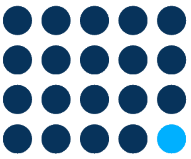99%### What characteristics of an investment affect risk?

All other things being equal, the more trades in an investment, the higher its risk.
All other things being equal, the higher the leverage and the longer the duration of the trades, the higher the risk.
• Market volatility and correlation of assets.
The higher the volatility and the correlation of the assets traded, the higher the risk.

### Where to check and track risk on the Darwinex platform

• On the DARWIN page.
DARWIN assets have a maximum monthly VaR of 6.5%, which is managed by our Risk Engine.
The monthly target VaR of the underlying trading strategy will most probably be different from the VaR of the DARWIN.

### Is it possible for investors in DARWINs to take less risk than 6.5% monthly VaR?

Yes, they can do this through diversification.

The more they diversify a portfolio among different DARWINs, the less the risk of your portfolio as a whole.

Portfolio risk can be seen in the "Investment"/"Portfolio risk" section.